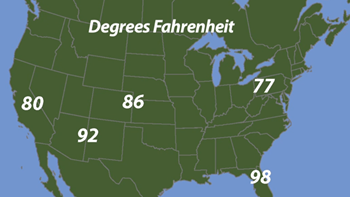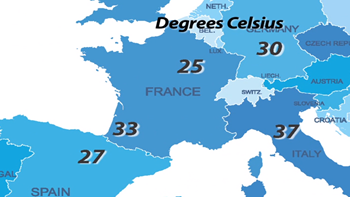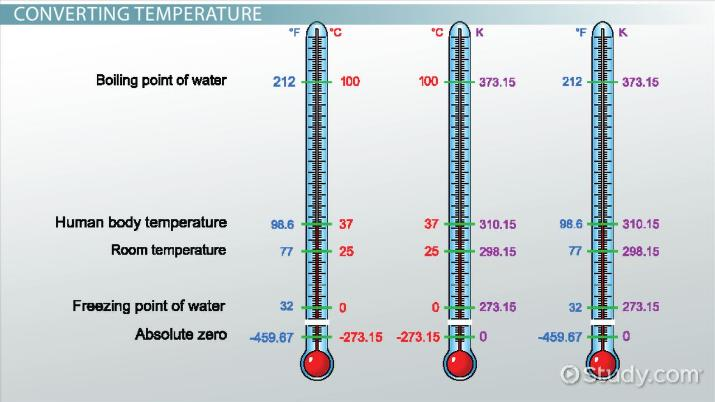Measuring Temperature & Converting Units of Temperature

Lesson Transcript
Instructor: Nicola McDougal

Nicky has taught a variety of chemistry courses at college level. Nicky has a PhD in Physical Chemistry.

Understanding temperature is important in daily interactions like choosing weather-appropriate attire or knowing how hot or cold something is. Explore how temperature is measured, how a thermometer works, and how to convert temperature from Fahrenheit to Celsius to Kelvin. Updated: 11/02/2021

Temperature and Weather Forecasts

One of my favorite activities is watching the weather forecast on TV. I am, like many other people, obsessed with what the weather is going to do. Will it snow? Will it rain? Will it be sunny? How hot will it be?

Here in the USA, we still use the degrees Fahrenheit scale for weather forecasts. But, in many other places in the world, weather temperature is measured using the degrees Celsius scale.The map on the left of the screen is showing various city temperatures ranging from 77 to 98 degrees Fahrenheit. On the map on the right, the temperatures are shown between 25 and 37 degrees Celsius. It is important that you can convert between these two units easily. We will learn how to do this a little further into this lesson.An error occurred trying to load this video.

Try refreshing the page, or contact customer support.

Coming up next: Mean, Median, Quartile, Range & Climate Variation of Temperature

You're on a roll. Keep up the good work!

Replay
Your next lesson will play in 10 seconds
• 0:05 Temperature
• 0:53 Measuring Temperature
• 1:52 Units of Temperature
• 3:13 Converting Temperature
• 7:10 Lesson Summary
Save Save

Want to watch this again later?

Timeline
Autoplay
Autoplay
Speed Speed

Measuring Temperature

Temperature can be simply defined as a measure of hotness or coldness of an object. It is always in reference to a temperature scale.

Temperature is measured using a thermometer. When we use a thermometer, we are observing the effect of temperature on the substance inside it. In the old days, thermometers were made of mercury in glass. As the temperature got hotter, the mercury expanded and moved up a narrow tube. We could see what the temperature was by reading it off the scale of numbers on the tube.

Mercury is no longer used in thermometers because it is very toxic. Modern thermometers use a different liquid instead, but the concept is still the same. As the temperature goes up, the liquid expands and rises up the tube. Of course, the reverse is true because when the temperature goes down, the liquid shrinks and drops down the tube.

Units of Temperature

We already mentioned two commonly used temperature units at the beginning of the lesson. In the USA, we still use the old Fahrenheit scale. Using this scale, the normal freezing point of water is 32 degrees F, and the normal boiling point of water is 212 degrees F.

But, outside of the USA and in many science applications, you will likely use the Celsius scale. This time, the normal freezing point of water is 0 degrees C, and the normal boiling point of water is 100 degrees C. Quite a difference in range! Now, you may have heard of the term 'degrees Centigrade.' If you have, then don't worry; that isn't something new. That is the same as degrees Celsius; they just changed the name from Centigrade to Celsius.

The final temperature unit you may come across is called the Kelvin. Note it isn't degrees Kelvin, just plain Kelvin. We certainly wouldn't forecast the weather using Kelvin, but it is very useful in many physics and chemistry applications. It is mainly used because the Kelvin scale has no negative numbers. The lowest temperature any substance can drop down to is 0K, or absolute zero.

Converting Temperature

Now that we have met the three different units of temperature, let's learn how to easily convert between them. Let's begin by learning how to convert from degrees Celsius to degrees Fahrenheit.

Converting from Degrees Celsius to Degrees Fahrenheit

Before we dive into the math, it is good to get a feel for the sort of number you are looking for. I remember a little rhyme I was taught at school when trying to figure out the conversions between Fahrenheit and Celsius:

• In degrees Celsius, the 30s are hot, 20s are pleasing, 10s are not, and 0 is freezing.

So, now that we know the sort of number we are expecting, let's do the math.

The temperature in Indianapolis will be 31 degrees Celsius today. What is this in degrees Fahrenheit?

Okay, so based on our little rhyme, we know that 31 degrees Celsius is hot. So, we expect a temperature in Fahrenheit to be well in the 70s on the Fahrenheit scale. The conversion factor we use to convert from degrees Celsius to degrees Fahrenheit is the temperature in Fahrenheit is equal to 1.8 multiplied by the temperature in Celsius plus 32:

TF = 1.8TC + 32

Let's plug in the numbers:

TF = 1.8 x 31 degrees C + 32

TF = 87.8 (or 88) degrees Fahrenheit

Yes, that looks pretty hot to me!

Converting from Degrees Fahrenheit to Degrees Celsius

Now we can do a very similar calculation if we want to convert from degrees Fahrenheit to degrees Celsius.

To unlock this lesson you must be a Study.com Member.

Register to view this lesson

Are you a student or a teacher?